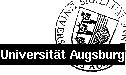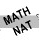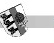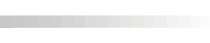Universität Augsburg - Institut für Physik## Computational Physics

Contents

• numerical accuracy, errors, and limitations
• linear algebraic equations
• interpolation and extrapolation
• differentiation and integration
• evaluation of functions
• special functions (Bessel functions, spherical harmonics)
• random numbers
• eigensystems
• root finding, nonlinear equation systems
• optimization (genetic algorithms, simulated annealing)
• spectral analysis (Fourier transform)
• modelling of data
• ordinary differential equations
• integral equations (maximum entropy method)
• partial differential equations
• finite element methods
• Monte-Carlo techniques
• lattice summation techniques (Ewald method, fast multipole method)
• Brillouin zone integration techniques

Requirements: Basic courses in mathematics; a programming language (Fortran95, C)

LiteratureVolker Eyert's Homepage EP VI Last revision: 17.01.2006 ©1998-2023 Volker Eyert Disclaimer